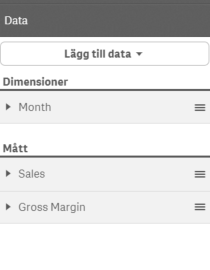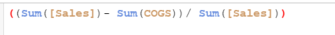Qlik Sense App Development

Discussion board where members can learn more about Qlik Sense App Development and Usage.

HighlightedNot applicable

Custom color measurements

Hello everybody,

Here comes another color question. I have a chart that looks like this:I have 1 dimension and 2 measures:Gross margin is calculated like this:(for those who want to know).

I would like to be able to custom color my measure (Gross Margin). Can anyone tell  me how to do it?

Sincerely,

Betty Habtemariam

Tags (2)
2 Replies
Valued Contributor II

Re: Custom color measurements

Hi Betty

May be somethink like this :

ColorMix1(

((Sum(Sales)-sum(COGS))/Sum(Sales)-\$(=min(aggr( ((Sum(Sales)-sum(COGS))/Sum(Sales), [Month])))))

/

\$(=(max(aggr(((Sum(Sales)-sum(COGS))/Sum(Sales),[Month]))-min(aggr(((Sum(Sales)-sum(COGS))/Sum(Sales), [Month])))))),white(),blue())

Hope it helps

Bruno

Honored Contributor II

Re: Custom color measurements

Hi Betty,

As you have the gross margin as one of he measures , you will be able to colour code the chart with just an if statement like this:

if( (sum(sales)-sum(cogs))/sum(sales) > 0.5,green(), // greater than 50% green

if( (sum(sales)-sum(cogs))/sum(sales) < 0.2,red(), // less than 20% red

blue())) // else blue

Thanks,

Sangram